# Adding new operations to DRC engine

I am working on a project that requires using some DRC operations that aren't provided by KLayout DRC engine. For example, I need operations that select edges based on their orientation, e.g. "select edges from layer1 and layer2 that coincide and have the interior of the corresponding polygon on the same side". I could probably do some hack that includes extended_in/extended_out operations, but I would like to know how to add operations to the engine in general. I understand that it won't be simple, but if someone could point me in the right direction I would be extremely grateful.

Regards,
Mikhail

• Hi Mikhail,

please consider the DRC features as a kind of tool kit. You will find generic tools, but you have to use your intuition to use them to combine them into complex features.

If I understand your request correctly, the following code should do the job:

``````layer1 = ...
layer2 = ...

both = layer1 & layer2
edges_of_both = both.edges
result = layer1.edges & layer2.edges & edges_of_both.edges
``````

The idea is to compute the intersection (AND). Edges on this part coinciding with original edges must be edges where the originals coincided that had their inner part on the same side (otherwise AND is empty).

I feel that are even simpler solutions, but this was the first one to come up with.

Matthias

• Thank you Matthias. This code indeed does what I need for this example. However, I would like to know how to work with the DRC engine in general, i.e. not combining existing operations but writing brand new ones (presumably using C++). Could you please tell me where to look for low-level implementations of existing operations so I can better understand how the layer operations work down to the lowest level?

Regards,
Mikhail
• Hi Mikhail,

it is definitely not an easy task and you will need support to achieve this. There are several engines (flat and hierarchical) and the concepts are not trivial.

I'd like to know what you're planning. What specifically are you trying to do? And is that going to be some open source feature? Honestly, I do not wish to waste my time on private extensions. However, if you plan to build a feature which is supposed to be merged back into the public domain, I am willing to give you some support.

Matthias

• Hi Matthias. I am trying to write DRC scripts for a coulpe of PDKs using open-source tools. I have Calibre SVRF scripts to base my scripts on, but they use some operations that are have no analogs in the KLayout format (e.g. PATH LENGTH operation). The example that I gave in the first message was COINCIDENT INSIDE EDGE operation from SVRF, which I thought would be problematic to do in KLayout because of the differences of edge direction representation.

The idea is to add operations to the engine that would cover the most used SVRF operations in terms of functionality. All new operations will be open-source.

Regards,
Mikhail

• You should be super careful to not simply duplicate concepts from SVRF. They may be patented features and SVRF is protected by the NDA clause you have signed together with the Calibre license agreement. I personally will not commit to anything that targets "SVRF compatibility".

But after all, the SVRF deck is only one of many possible implementations of the design rules in your PDK. There is always some idea behind a rule which is worth elaborating. Let's rather think of generic atomics you can build your solution from. Simple SVRF compatibility cannot be a design goal under the given circumstances and I see that as an opportunity rather than a limitation.

Maybe you can give some explanation for the checks you're trying to map and we can discuss alternative features to support them.

Matthias

• One of the SVRF operations that I encounter frequently is `ENCLOSE RECTANGLE`, which selects polygons that can fit a rectangle with certain dimensions. Suppose a metal normally has minimal spacing `S1`, but the minimal spacing between two metal polygons that have parallel run length greater than some `L` must be `S2 > S1`. I've seen this done in SVRF in a following way: first we get the region between the metal polygons where spacing is less than `S2` (light blue on the first picture) using something like `X = EXT metal < S2 REGION`, then we try to fit into this region a rectangle with length L and width equal to the grid (i.e. `ENCLOSE RECTANGLE X GRID L`). Another way of doing this is selecting the edges as shown with blue color in the second picture and applying `PATH LENGTH` operation to them, thus selecting the edges which are part of a chain with length `>L`.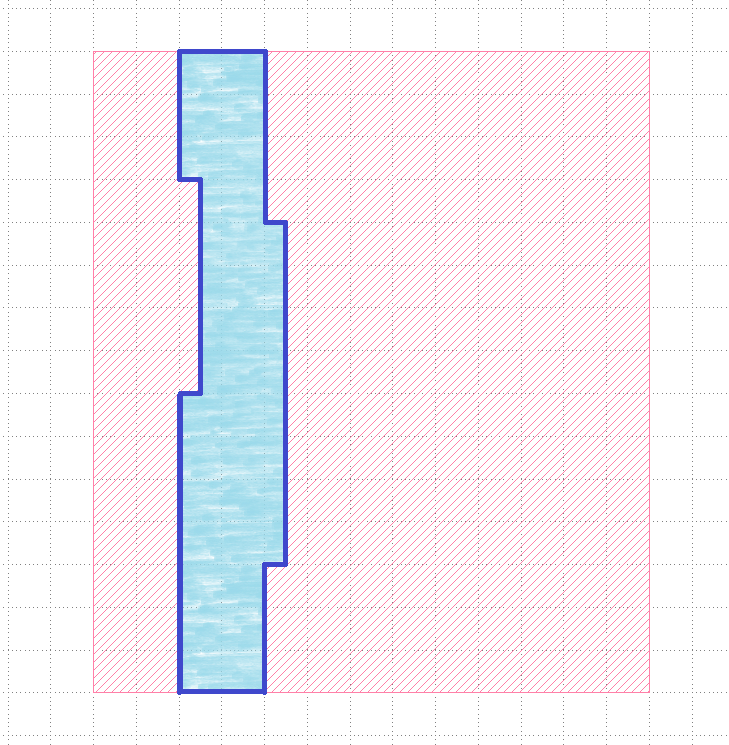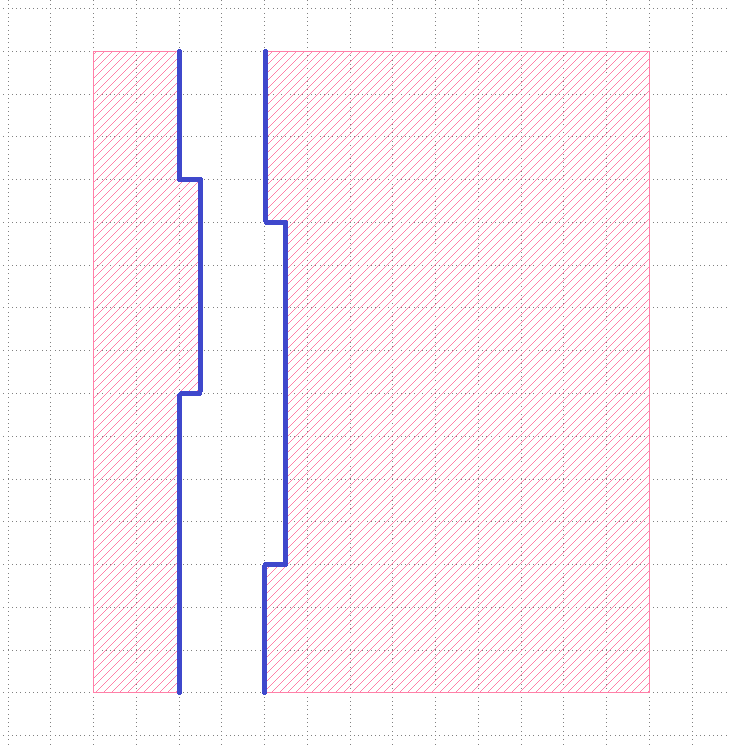This particular situation can be handled adequately with KLayout, but that's not the point. I think "select all polygons on a polygon layer that can fit an `X` by `Y` rectangle" and "select all edges on an edge layer that are a part of a polygonal chain that is longer than `L`" are useful operations. Do you think they could be added to KLayout DRC operations with reasonable effort?

Regards,
Mikhail

• edited September 10

Hi Mikhail,

thank you for that explanation. This is already very useful.

The valuable piece of information is the description of the rule. That makes sense physically (likelihood of defects increased with run length, lithography will have more trouble like increased risk of side lobe printing or resist stability).

So to summarize the spec:

• Min space S1 when common run length is < L
• Min space S2 > S1 when common run length is >= L

There is an option already which maybe implements these checks. It is "projecting" and it allows confining the check to situations where the projection of the involved edges satisfies a certain condition.

Here is an example:

``````report("Discussion 2141")

L = 2.um
S1 = 1.1.um
S2 = 1.6.um

layer1 = input(1, 0)

layer1.space(projection, projecting < L, S1).output("space < #{S1} for common run length < #{L}")
layer1.space(projection, projecting >= L, S2).output("space < #{S2} for common run length >= #{L}")
``````

With this sample layout: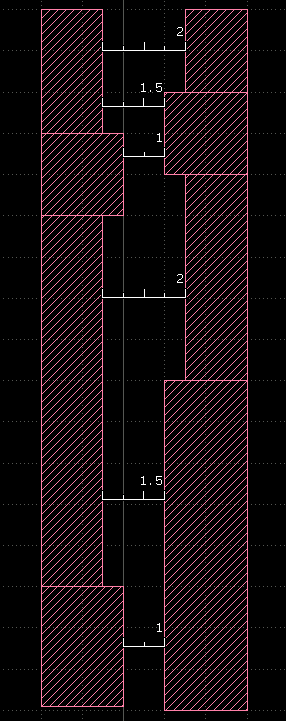I get these results:

First check: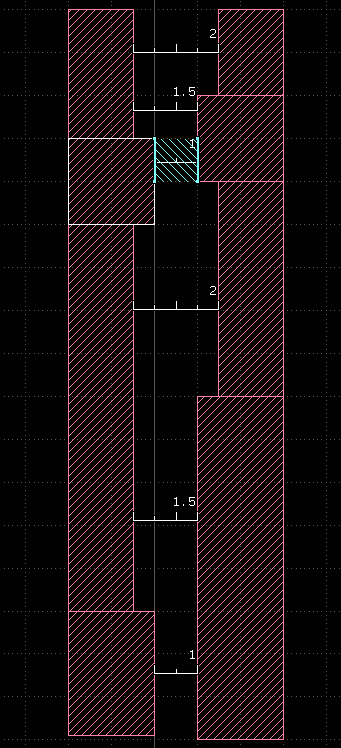Second check: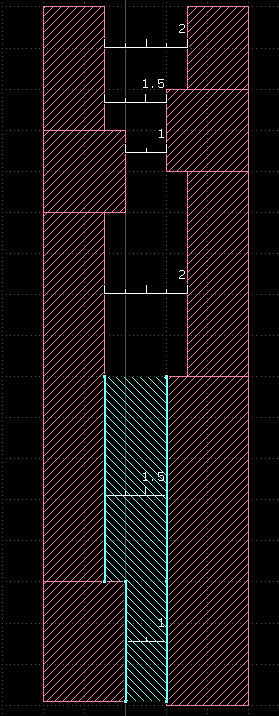If you insist on "select regions which fit and x by y rectangle", this function can be emulated:

``````class DRC::DRCLayer
# Returns the parts of self where a box with dimension l x w can be fit.
# Both possible orientations of the box are considered.
# This implementation works for Manhattan geometries.
def encloses_box(l, w)
sl = -0.5 * l + 1.dbu
sw = -0.5 * w + 1.dbu
if (sl - sw).abs > 1e-6
return self.sized(sl, sw).sized(-sl, -sw).or(layer.sized(sw, sl).sized(-sw, -sl))
else
# optimized case for square
return self.sized(sl).sized(-sl)
end
end
end

layer1 = input(1, 0)

layer1.encloses_box(2.um, 2.um).output(1000, 0)
``````

This will monkey-patch the layer class to supply a new feature which returns all parts that enclose the given box. The implementation uses under/oversize to select parts with a big enough size for the box. Two steps are required to fit the box in both possible orientations unless the box is a square.

Beware that this version only works for Manhattan geometries. A general version is this:

``````class DRC::DRCLayer
# Returns the parts of self where a box with dimension l x w can be fit.
# Both possible orientations of the box are considered.
# This implementation works for any-angle geometries.
def encloses_box(l, w)
sl = -0.5 * l + 1.dbu
sw = -0.5 * w + 1.dbu
if (sl - sw).abs > 1e-6
r0 = self.sized(sl, 0).sized(-sl, 0).sized(0, sw).sized(0, -sw)
r90 = self.sized(sw, 0).sized(-sw, 0).sized(0, sl).sized(0, -sl)
return r0.or(r90)
else
# optimized case for square
return self.sized(sl, 0).sized(-sl, 0).sized(0, sl).sized(0, -sl)
end
end
end

layer1 = input(1, 0)

layer1.encloses_box(2.um, 2.um).output(1000, 0)
``````

Here is the result: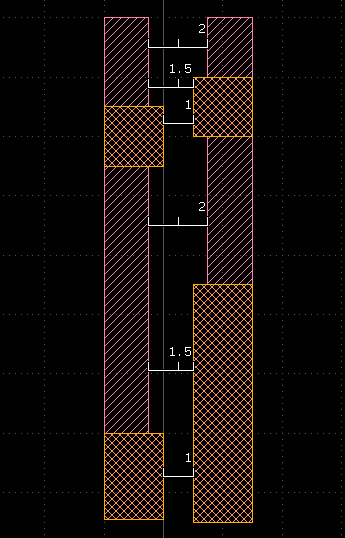And finally about the "select edges which are part of a chain with length L": A straight chain of edges can be length-selected in KLayout using "with_length". KLayout merges edges before feeding them into the length measurement algorithm. Hence you can put in disconnected edges too. Here is an example:

``````layer1 = input(1, 0)

# selects edges with L >= 4 µm
layer1.edges.with_length(4.um, nil).output(1000, 0)
``````

which gives: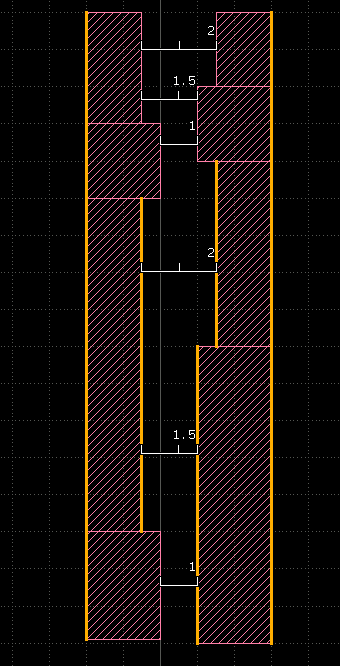Matthias